# IFS Attractors: Polyominos

Polyominoes are a set of mostly irregular polygons which are constructed by joining squares together at a common edge. The number of polyominos increase rapidly with the number of squares in the polyomino, there being 1 monomino, 1 domino, 2 triominos, 5 tetrominos, 12 pentominos, 35 hexominos, 108 heptominos, etc.

All members of two classes of polyominos - the rectangular and rectifiable polyominos - are rep-tiles.

The rectangular polyominos, being parallelograms, all have rep-number 4 (or in general k2).

Rectifiable polyominos are those which can tile a rectangle. As the ratio of the sides of such rectangles is rational, and as any rational sided rectangle can tile any polyomino, there is a construction by which and IFS can be generated for any rectifiable polyomino. In at least some cases (L-triomino, P-pentomino) there are dissections with smaller rep-numbers. In many cases they are irreptiles with smaller numbers of elements.

I am not aware of any non-rectangular, non-rectifiable, polyomino, which is an rep-tile, but at least one is an irrep-tile.

The rectangular polyominos, being rectangles, are rep-tiles with a rep-number of 4. Rectangular polyominoes include the monomino, domino, I-triomino, I-tetromino, O-tetromino, I-pentomino, I-hexamino, O-hexamino, etc. If n is prime there is one rectangular n-omino. If n is composite, there is more than one rectangular n-omino. In particular there are 2 rectangular octominos, 2 rectangular decominos, 3 rectangular dodecominos, 2 rectangular tetradecominos, 2 rectangular pentadecominos, 3 rectangular hexadecominos, 3 rectangular octadecominos, 3 rectangular eicosominos, 2 rectangular uneicosominos, 2 rectangular doeicosominos and 4 rectangular tetraeicosominos.

All rectangular polyominos tile the plane with a single copy per unit cell.

 Polyomino IFS Name Min # in unit cell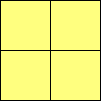monomino square 1domino 2:1 rectangle 1I-triomino 3:1 rectangle 1I-tetromino 4:1 rectangle 1O-tetromino square 1I-pentomino 5:1 rectangle 1I-hexomino 6:1 rectangle 1O-hexomino 3:2 rectangle 1

As they are rectangles, rectangular polyominos also have rep-number n2. Some I-polyominoes reappear as rep-n rectangles, e.g. the domino is the rep-4 rectangle, the I-triomino the rep-9 rectangle, and the I-tetromino the rep-16 rectangle.

"Irregular" constructions are known for the L-triomino and P-pentomino. It is likely that they exist for other polyominoes, but I have no evidence that this is the case. It can fairly readily be seen that no low rep-number "irregular" construction exists for the T-tetramino, and that the S/Z-tetromino and X-pentomino are not rep-tiles.

Both the L-triomino and the P-pentomino are rep-4 tiles. The L-triomino is also a rep-9, rep-16, rep-25, rep-36, rep-49, rep-64, rep-81, rep-100 and rep-144 tile, and I conjecture that it (like the triangle, parallelogram and triamond) is a rep-n2 tile.

All constructions for the L-triomino are special cases of a continously variable set of rep-tiles, with a single degree of freedom. These sets also include the P-hexomino (a tri-domino), a tri-I-triomino, a tri-I-tetromino, etc. (Note: This requires that the different orientations are achieved by means of reflections, and not by rotations.)

 Polyomino IFS Name Min # in unit cell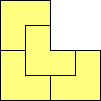L-triomino (rep-4) 2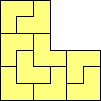L-triomino (rep-9) 2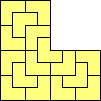L-triomino (rep-16) 2P-hexomino tridomino 29-omino tri-I-triomino 2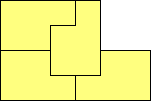P-pentomino 2 4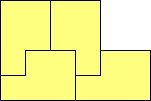P-pentomino 2 4

A rectifiable polyomino is one which can be tiled to create a rectangle. The following construction can be used to create an IFS for a rectifiable polyomino.

1. k copies of the polyomino can be tiled to create a rectangle.
2. m copies of the rectangle can be tiled to create a square.
3. n copies of the square can be tiled to create the polyomino.

This construction generates a rep-kmn polyomino. There are often different possible values for k. The same construction can be used for rectangular polyominos, with k=1.

Lower rep-number IFSs can sometime be generated by replacing the square in steps 2 and 3 above by a domino. This can only apply to even polyominos, and only to those even polyominos can can be dissected into dominos. Examples are the L-tetromino, P-hexomino and J-hexomino. In principal this can also be applied with triominos, tetrominos, etc, but examples are infequent.

Tilings of rectangles by polyominoes can be found at M. Reid's page at the University of Massachussets.

 Polyomino IFS Name Min # in unit cell Rep-numberL-triomino 2 36L-tetromino 2 16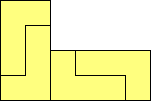L-tetromino L-didomino 2 4T-tetromino 4 16L-pentomino 2 100P-pentomino 2 100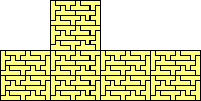y-pentomino 10 100L-hexomino 2 36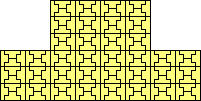D-hexomino 4 144U-hexomino 2 144A-hexomino 2 144P-hexomino 2 144P-hexomino P-tridomino 2 36J-hexomino 2 144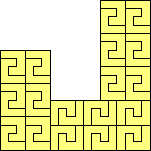J-hexomino J-tridomino 2 36G-hexomino R-hexomino 18 1296y-hexomino 480 14400

There is a rep-144 implementation of the P-hexomino, with a 4x3 unit cell, rather than the 6x2 unit cell shown above.

The IFSs for the G/R- and y-hexominos are not shown as their rep-numbers are so high they cannot be clearly displayed at the image size used. (Never mind the work writing down the IFSs.) There is a version of the y-hexomino with 92 copies per unit cell. This however is rep-304704.

The following are not rectifiable, and either have no IFS, or are not known to have an IFS.

 Polyomino Name Min # in unit cell Rep-numberZ-tetromino no IFSN-pentomino no known IFST-pentomino no known IFSU-pentomino no known IFSV-pentomino no known IFSW-pentomino no known IFSX-pentomino no IFSZ-pentomino no IFS

Order-2 rectifiable polyominos are special cases of a continously deformable sets of rep-tiles with two degrees of freedom. Other special cases of these sets include polydiamonds, polydiabolos (polyditans) and polydidrafters. For example the rep-36 L-triomino set also includes the lobster and chevron hexiamonds, and the corresponding hexabolos and hexadrafters.

Mirror-symmetric order-4 rectifiable polyominoes are special cases (with the mirror image used for the vertical placed elements) of continuously deformable sets of rep-tiles. For example the rep-16 T-tetromino set also includes 2 octiamonds. I have yet to investigate whether these sets have one or two degrees of freedom. I have yet to investigate which, if any, non-mirror-symmetric order-4 rectifiable polyominoes are members of continously deformable sets.

The order-10 y-pentomino, order-18 G-hexomino and order-480 y-hexomoino are not continuously deformable; I conjecture that this holds for all rectifiable polyominoes with orders other than 2 or 4.

Sources: The IFSs for rectifiable polyominoes are independently invented (I assume that these are well-known, as the follow from the widely studied problem of tiling a rectangle with a individual polyomino) except that the tilings of a rectangle from which the IFSs for the y-pentomino and R/G- and y-hexominos were taken from M. Reid's page at the University of Massachussets. The rep-4 implementation of the L-triomino is independently invented, though I have seen this elsewhere since, as are the related rep-4 implementations of the P-hexomino and subsequent tri-I-polyominos. The 1st rep-4 implementation of the P-pentomino and the rep-9 implementation of the L-triomino were taken from Eric Weisstein's World of Mathematics. The 2nd rep-4 implementation of the P-pentomino is taken from A.L. Clarke's PolyPages

References

© 2001, 2002, 2007 Stewart R. Hinsley